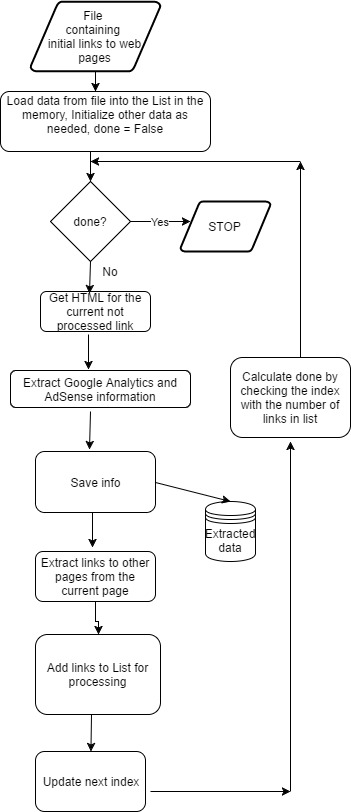Recently I decided to get information that is showing for each page of my website Google Analytics account number and all Google AdSense links on this page. Connecting this information with Google Publisher Pages data would be very useful for better analysis and understanding of ads performance.

So I created python script that is doing the following:

1. Opens file with some initial links. The initial links then are extracted into the list in computer memory.
3. Extracts Google Analytics account number from HTML. The acconneunt number usually appears on web page code on the line, formatted like this : ‘_uacct = “UA-xxxxxxxxxx”;’ The script extracts UA- number using regular expression.
4. Extracts Google AdSense information from HTML text. AdSense information is displayed within /* */ like below:

/* 300×15, created 1/20/17 */
Here ‘300×15, created 1/20/17’ is default ad name.

5. Extracts all links from the same HTML text.
7. Outputs extracted information to csv file. The saved information contains url, GA account number and AdSense ad names that are on the page.
8. Repeats steps 2 – 6 while there are links to process.

Here are few examples how the script can be used:

• Lookup of page for the given ad. For example AdSense is showing clicked link and we want to know what page it was.
• Check if all pages have GA and AdSense code inserted.
• Use the data with Google Analytics and AdSense for analysis of revenue variation or conversion rate by ad size for different group of web pages.

Below you can find script source code and flow chart.

``````
# -*- coding: utf-8 -*-

import urllib.request
import lxml.html
import csv
import time
import os
import re
import string
import requests

path="C:\\Users\\Owner\\Desktop\\2017"

filename = path + "\\" + "urlsB.csv"

filename_info_extracted= path + "\\" + "urls_info_extracted.csv"

urls_to_skip =['search3.cgi']

start=0
file_urls=[]
with open(fn, encoding="utf8" ) as f:
for i, row in enumerate(csv_f):
if i >=  start  :
file_urls.append (row)
return file_urls

def save_extracted_url (fn, row):

if (os.path.isfile(fn)):
m="a"
else:
m="w"

with open(fn, m, encoding="utf8", newline='' ) as csvfile:
fieldnames = ['url', 'GA', 'GS']
writer = csv.DictWriter(csvfile, fieldnames=fieldnames)
if (m=="w"):
writer.writerow(row)

print ("Starting navigate...")

url_ind=0
done=False
while not done:
u=urlsA[url_ind]
new_row={}
print (u)
print (u)

try:
connection = urllib.request.urlopen(u)
print (u)
print ("connected")
time.sleep( 12 )
r = requests.get(u)

# Get the text of the contents
html_content = r.text

pat = re.compile(r"(/\*(.+?)\*/)+", re.MULTILINE)
if pat.search(html_content) != None:

str=""
for match in pat.finditer(html_content):
print ("%s: %s" % (match.start(), match.group(1)))
str=str+","+ match.group(1)
new_row['GS'] = str

pat1 = re.compile(r"_uacct(.+?)\"(.+?)\"", re.MULTILINE)
if pat1.search(html_content) != None:

m=pat1.search(html_content)
new_row['GA'] = m.group(2)

new_row['url'] = u
save_extracted_url (filename_info_extracted, new_row)
url_ind=url_ind+1

print (html_content)

if "lwebzem" in a:

try:

if ind >=0:
else :

except :

skip = False
skip=True
skip = True